# Time Series Forecasting: The Most Basic Models

## What is Time Series Forecasting?

A time series is a collection of observations made sequentially through time, e.g. the value of a company’s stock. Time series forecasting is the practice of making predictions based on a time series. That is, if we’re given some time series data,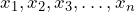our goal is to predict the value of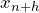, where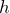is known as the forecast horizon or lead time.

## Time series models

In the models below,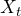is a value in the time series,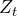is a value from a purely random process with 0 mean and constant variance, and the greeks represent the parameters that are learned when training the model.

### The random walk

The random walk model is a model in which values in a time series are taken to be the most recent value with some random error tacked on. That is,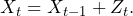The implications of a random walk process are that its values cannot be predicted since it is impossible to predict the next value in a purely random process. Many believe most financial data follow a random walk. This is debatable however and there has been evidence that the complexity of financial data causes inaccurate predictions but that it is still predictable.

### Autoregressive Processes

A times series is said to be an autoregressive process of order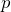, abbreviated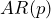if the values of the time series are taken to be a weighted linear sum of themost recent values with noise.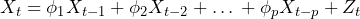### Moving Average Processes

Like the autoregressive process, the moving average takes into account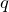of the most recent values in the model. Unlike the autoregressive process, the moving average process takes into accounterror terms rather than time series values as well as the mean of the time series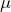. That is, the moving average process of order,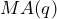says,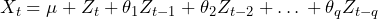In most situations, the mean of a time series is assumed to be 0. Thus the model above becomes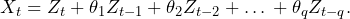### Autorgressive Moving Average Processes

The next two models essentially take the previous two models and shove them together. The first model, the autoregressive moving average (ARMA) model of order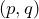does exactly that. The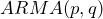model combines anmodel and anmodel to get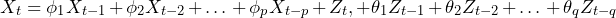making values in the time series dependent on past time series values as well as past error terms.

### Autoregressive Integrated Moving Average Processes

The autoregressive integrated moving average process (ARIMA) of order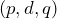is anmodel that is fit after some modifications to the data are performed. These modifications make non-stationary data, which is data whose statistical properties (mean, variance, autocorrelation structure, etc.) change over time, to stationary data which is data with constant statistical properties. One technique for making non-stationary data stationary is differencing the values of the times series so that,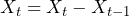if the data is differenced once, or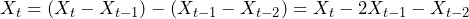if the data is differenced twice, and so on for as many iterations required before the data becomes stationary. This brings us to our definition of the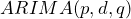model. Themodel is anmodel that is fit to data after it is differenced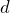times. In essence, the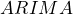model provides a way to handle more complex datasets with the models above since these methods assume the data is stationary. In practice, most time-series data is not stationary so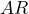,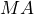and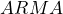processes cannot be applied directly, making anmodel the model of choice in most situations.

## Implementations in R

Introduced above are some basic models used for time series forecasting which are used as the foundations of many more algorithms and models. However, many of the models above are rarely (directly) used since themodel can be used to represent any of the others. Consider the case of themodel. An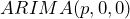model yields the same result. Forandmodels the correspondingmodels are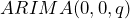and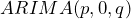, respectively. Even the random walk model can be modeled via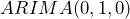. This will be a useful fact in the following R implementation.

### The Dataset

The dataset I’ll be using with these methods is historical data of the EUR/USD foreign exchange rate. This dataset consists of the closing prices of the exchange rate between January 1, 1999 and November 30, 2017 which provides 4,935 samples to be split between training and testing. Predicting foreign exchange rates is an important task in economics, finance, and business but, due to the complexity of the data, most methods perform poorly on out-of-sample data when compared to a simple random walk model. A plot of this dataset is shown below.

### Setup

Before training any models we will need to read in the dataset and split it into training and testing subsets. In this case, we will predict one timestep (day) into the future and re-train the models after each prediction. Therefore, a small subset of data (e.g. 10 samples) is used to train the initial model and a history of points is kept for subsequent training. However, the data used to train the model will always be 10 samples so the first (oldest) data point is dropped from the training data and the new, previously unseen data point is added to the training data. The following R code get’s the ball rolling.

data <- read.csv("eurusd.csv")   # read in the data
xy <- data$EUR.USD # get only the time series values train <- xy[1:10] # get the initial training data test <- xy[11:length(xy)] # store the remainder of the data in the test vector dates <- data$Date[11:length(data$Date)] # for visualizing results Using 10 samples is chosen somewhat arbitrarily. Therefore the number of samples used to fit the models can vary. ### ARIMA It may seem counterintuitive to start with the ARIMA model since it is built out of the other models. The reason for doing so will become apparent when implementing the more basic time series models. Fortunately, the stats package included with R already has the ARIMA model built-in. Thus the implementation of ARIMA in R is as follows, arima <- function(p, d, q, data) { optim.control = list(maxit=2000) return(stats::arima(data, order=c(p, d, q), method="ML")) } This function will return an ARIMA model object from the stats package, i.e. stats::arima(). The model will have been trained from the data passed into the function. Below, I will explain how to use the model to get one-step-ahead predictions with retraining at each timestep. There are two things to note here: the first is that we’re increasing the max number of iterations to hopefully get better convergence of the methods and the second is that we’re forcing ARIMA to use Maximum Likelihood Estimation (“ML”). The latter causes the method to be slower but produces better results and always returns a stationary model. ### All Other Models Since the ARIMA model can model any of the others the ARMA, AR, MA, and Random Walk models are easily implemented with the ARIMA function above. #### ARMA arma <- function(p, q, data) { return(arima(p, 0, q, data)) } #### MA ma <- function(q, data) { return(arma(0, q, data)) } #### AR ar <- function(p, data) { return(arma(p, 0, data)) } #### Random Walk random_walk <- function(data) { return(arima(0, 1, 0, data)) } As shown above, each model can be created by delegating the construction to either the ARIMA method directly or indirectly via the ARMA method. ### Using the Models Now that the models have been created and fit to existing data, we can use the remainder of the data to get predictions and retrain the model as we go. The following R code does just that. The first function is used to train the model depending on the parameters passed into the main predict method. The implementation below is a little bit sloppy but it works fine. train_model <- function(model, order, train) { mdl <- NULL if(model == "ar") { mdl <- ar(order, train) } else if (model == "ma") { mdl <- ma(order, train) } else if (model == "arma") { mdl <- arma(order, order, train) } else if (model == "arima") { mdl <- arima(order, order, order, train) } else if (model == "rw") { mdl <- random_walk(train) } return(mdl) } Depending on what the user specifies in the predict function (below), the method above will train the appropriate model. Below is the predict method which iterates through each value in the testing dataset and predicts the next value in the time series. Afterwards, the history vector is updated and the model is re-trained. predict <- function(model, order, train, test) { if(!(model %in% c("ar", "ma", "arma", "arima", "rw"))) { print("Invalid model specified.") return() } library(forecast) # for predictions hist <- train[-1] # create history of previous points, removing oldest mdl <- train_model(model, order, train) # train initial model if(is.null(mdl)) { print("Error creating model.") return() } predictions <- c() # store predicitons for(i in 1:length(test)) { # get predicition predictions <- c(predictions, forecast(mdl, 1)$mean[])

# get new training data
hist <- c(hist, test[i])
hist <- hist[-1]
# train new model with new data at each time step
mdl <- train_model(model, order, hist)
if (is.null(mdl)) {
print("Error creating model.")
return()
}
}

# for plotting
return(predictions)
}

### Results

This section contains some results from running the code above. I won’t run through each model since that would make this post too long but I will demonstrate a few different models. Before I do that, I have to define a function that was created to quickly and easily display the results, in case you wanted to create these visualizations yourself.

display <- function(predictions, actual, dates, points=NULL) {
if(length(predictions) != length(actual) || length(actual) != length(dates)
|| length(predictions) != length(dates)) {
print("Invalid prediction, dates, and actual vectors")
return()
}
if(is.null(points)) {
points <- length(predictions)
}

# plot predicted values
plot(predictions[1:points]~as.Date(dates)[1:points], xlab="Date", ylab="EUR/USD", main="Results", col="orange", type="l", lwd=2)
lines(test[1:points]~as.Date(dates)[1:points], col="green")
# display the legend
legend("topright", legend=c("Predicted", "Actual"), col=c("orange", "green"), lty=1:1, cex=0.9)
}

This method is used to plot both the actual and predicted values.

The first model used was an AR(1) model. Using the functions above, the R code to run this method is as follows

preds <- predict("ar", c(1), train, test)
display(preds, test, dates, 100)

The first call actually runs through the test set with the AR(1) model and gets the predictions. The second call plots the first 100 predictions vs. the first 100 actual values. These calls produce the following visualization.

As shown here, the model doesn’t perform very well on the dataset. It’s a very simple model so that is to be expected. Now let’s try a more complicated ARIMA model. For no reason at all I’ll choose an ARIMA(5,1,3) model. Because this model has a longer autoregressive component, the training data needs to be updated to include more samples. The calls to redefine the train, test, and dates vectors, to run the algorithm and to display the results are

train <- xy[1:100]
test <- xy[101:length(xy)]
dates <- data\$Date[101:length(xy)]
preds <- predict("arima", c(5, 1, 3), train, test)
display(preds, test, dates, 100)

As was done previously, the first 100 predicted and actual values are plotted for comparison. Since more data was used for training, the comparison looks a little different from above.

As seen in the plot of the results, the ARIMA(5, 1, 3) model is considerably more accurate than the AR(1) model. The final model trained was a random walk (which has no parameters). Surprisingly, as seen in the graph below, the models does almost as well as the ARIMA(5, 1, 3) model.

## Conclusion

This concludes this introduction to some very basic time series forecasting models. Although details, such as uses for the models or the training of these models are excluded, this introduction provides an overview of the basic models used in time series forecasting, how they relate to each other, and their implementations in R. Using the logic above, you can play around with the R implementation on other datasets or with the EUR/USD dataset to try to get better results. It should also be noted that there are ways of determining optimal parameters for the ARIMA model. In R, the function call auto.arima(x) determines p, d, and q automatically. In future posts, I plan to discuss these models more in-depth and take a look at replacing them with more effective deep learning methods.